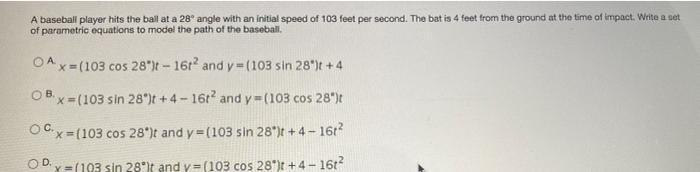Question:

# A baseball player hits the ball at a 28° angle with an initial speed of 103 feet per second. The bat is 4 feet from the ground at the time of impact. Write a set of parametric equations to model the pA baseball player hits the ball at a 28° angle with an initial speed of 103 feet per second. The bat is 4 feet from the ground at the time of impact. Write a set of parametric equations to model the path of the baseball. O A x=(103 cos 28°)t - 16t² and y=(103 sin 28°)t +4 O B x=(103 sin 28°)t +4-16t² and y=(103 cos 28°)t O C x=(103 cos 28°)t and y=(103 sin 28°)t +4-16t² O D x= (193 sin 28°)t and y=(103 cos 28°)t +4-16²